## Superconductivity Within a Slab

Original source of the model: F. Odeh: Existence and bifurcation theorems for the Ginzburg-Landau equations. J. Math. Phys. 8 (1967) 2351-2356.

Solutions are summarized in the branching diagram in Figure 1. A branch of asymmetric solutions branches off a branch of symmetric solutions at a pitchfork bifurcation point. The data of the bifurcation point and four turning points (TP) are listed in the Table.

Investigation of the above boundary-value problem reveals that it supports symmetry. To see this, define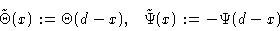and realize that the signs are the same after differentiating twice with respect to x. Hence,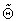and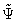satisfy the differential equations if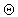and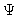do. Analyzing the boundary conditions, as, for example,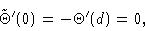shows thatandsatisfy the boundary conditions. Hence, the equation supports evenand odd. This does not imply that solutions must be even or odd. In fact, the equation has asymmetric solutions. See the solution in Figure 2, which depicts both the solutions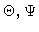and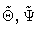for the parameter value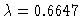.

In order to calculate solutions, we rewrite the boundary-value problem as a first-order system. Denoting,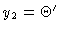,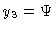, and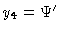yields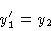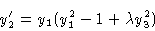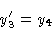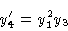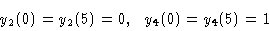The solution profiles of the bifurcation point are shown in Figures 4 and 5; notice the symmetries. This bifurcation point is straddled between the two turning points with asymmetric solutions, which are depicted in Figures 2 and 3. Compare the figures to see how this straddling becomes evident in the solution profiles.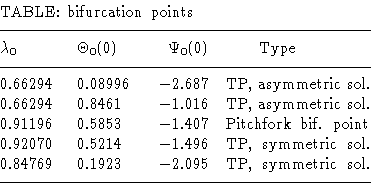Figure 1
Bifurcation diagram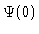versus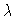. Two branches intersecting in a pitchfork bifurcation.

Figure 2
Two antisymmetric solutions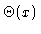for.

Figure 3
Two antisymmetric solutions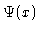for.

Figure 4
Symmetric solutionfor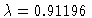.

Figure 5
Symmetric solutionfor.

This Example
Figure 1
Figure 2
Figure 3
Figure 4
Figure 5